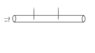# Pressure changes of a fluid flowing through a horizontal pipe

Photo1234
If we have a tube of fluid going like it is shown in the picture, and we measure pressure at two points we would get the same value. But the fluid is still going from point A to point B. So is pressure difference not necessary for fluid flow or I made some other wrong assumptions?

#### Attachments

•Slika nove bitne slike.jpg
9.5 KB · Views: 264

Homework Helper
So is pressure difference not necessary for fluid flow
Newton's first law. An object in motion will continue in motion at a constant velocity unless acted on by an outside force.

Staff Emeritus
Homework Helper
Gold Member
If friction and viscosity is significant you will need a pressure gradient to drive the flow. If they are not, the pressure in the two points will be the same.

•russ_watters and Chestermiller
Photo1234
What if we use electrons instead of fluid and use Ohms law V = R * I for two points like that, and If V = 0, that current should also be 0. Is that violation of Omhs law?
(We neglect resistance of the wire)

Staff Emeritus
What if we use electrons instead of fluid and use Ohms law V = R * I for two points like that, and If V = 0, that current should also be 0. Is that violation of Omhs law?
(We neglect resistance of the wire)

No it is not a violation and it does not need us to neglect the resistance. Let us say R=1, Then V=R*I means 0=1*0. Same answer if R=1000.

Staff Emeritus
Did you understand the answer in post #3?

Photo1234
So I = V/R = 0/0

And what causes current if not voltage? Inertia?

Mentor
So I = V/R = 0/0

And what causes current if not voltage? Inertia?
You're trying to find a physical meaning for an assumed - not real - mathematical result of 0/0. There really isn't one. Stick to the physical laws. Stick to the answer you got in post #3 and recognize that simplifications are sometimes useful, but sometimes give nonsensical results.

Photo1234
You're trying to find a physical meaning for an assumed - not real - mathematical result of 0/0. There really isn't one. Stick to the physical laws. Stick to the answer you got in post #3 and recognize that simplifications are sometimes useful, but sometimes give nonsensical results.

What abaut this:
And what causes current if not voltage? Inertia?

Staff Emeritus
Sorry, this question has been answered several times.